# Envelope

of a family of curves in the plane

The curve that at every point touches one of the curves of the family such that the points of contact along the envelope pass from one curve of the family to another. For example, the family of circles of the same radius with centres on a straight line has an envelope consisting of two parallel lines. Ifis the parameter of the family,is the parameter along the envelope and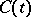the value offor one of the curves of the family touching the envelope at the point with parameter, then it is assumed that it is possible to choosesuch that the function is not constant on any part of the range of.

For the family of curves given by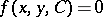, where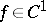and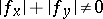, a necessary condition for the existence of an envelope is that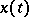,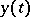,satisfy the condition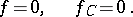(1)

The system (1) serves to determine the points of the envelope, but other singular points of the family may also satisfy (1). A sufficient condition for a point to belong to the envelope is that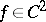and satisfies, in addition to (1), the condition(2)

For a family of plane curves given by a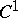-function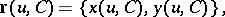whereis the parameter of the family andthe parameter along its curves, a necessary condition for a point to be on the envelope is that, or, which is the same thing,(3)

A sufficient condition is thatand that, in addition to (3),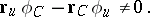(4)

Violation of conditions (2) and (4) is most often related to the appearance of cusps on the envelope.

The envelope of a family of surfaces in space depending on one parameteris the surface that at each of its points with intrinsic parameters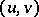touches the surface of the family with parameter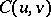, and is such that the functionis not constant on any domain in domain of definition of. For example, the envelope of spheres with the same radius and centres on a straight line is a cylinder. For a family given by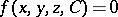, whereand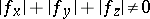, a necessary condition for the envelope is satisfaction of the system of equations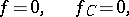(5)

and a sufficient condition is:and, in addition to (5), the conditions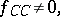(6)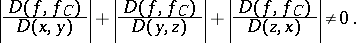For the family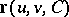, where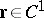and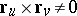, a necessary condition for the envelope is satisfaction of the equation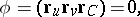(7)

and a sufficient condition is that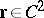and that, besides (7), the following conditions are satisfied: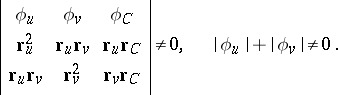(8)

Violation of the first of the conditions in (6) and (8) is most often related to the appearance of a cuspidal edge on the envelope. The line of contact of the envelope with one of the surfaces of the family is called a characteristic. A cuspidal edge on the envelope in turn is usually the envelope of the characteristics.

The envelope of a family of surfaces in space depending on two parametersandis the surface touching at each of its pointsthe surface of the family with parameters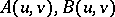, and such that there is no function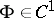on any domain of the range ofwith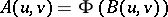. For the family given by the equation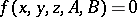, whereand, a necessary condition for the envelope is satisfaction of the system of equations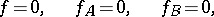(9)

and a sufficient condition is thatand that, as well as (9), the following conditions holds: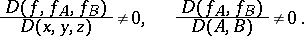For a family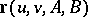, whereand, a necessary condition is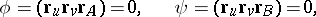(10)

and a sufficient condition is thatand, as well as (10), satisfaction of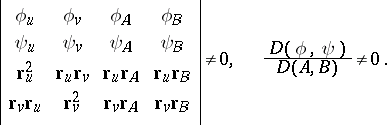The more complicated concept of the envelope of a family of-dimensional submanifolds depending onparameters in an-dimensional manifold can be introduced (see ) based on the theory of singularities of differentiable mappings as a special form of singularity of a family.